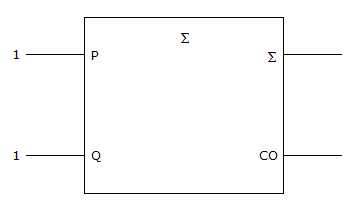# Digital Electronics - Digital Arithmetic Operations and Circuits

### Exercise :: Digital Arithmetic Operations and Circuits - General Questions

51.

Which of the statements below best describes the given figure?A. Half-carry adder; Sum = 0, Carry = 1 B. Half-carry adder; Sum = 1, Carry = 0 C. Full-carry adder; Sum = 1, Carry = 0 D. Full-carry adder; Sum = 1, Carry = 1

Answer: Option A

Explanation:

No answer description available for this question. Let us discuss.

52.

An 8-bit register may provide storage for two's-complement codes within which decimal range?

 A. +128 to –128 B. –128 to +127 C. +128 to –127 D. +127 to –127

Answer: Option B

Explanation:

No answer description available for this question. Let us discuss.

53.

A full-adder adds ________.

 A. two single bits and one carry bit B. two 2-bit binary numbers C. two 4-bit binary numbers D. two 2-bit numbers and one carry bit

Answer: Option A

Explanation:

No answer description available for this question. Let us discuss.

54.

The carry propagation delay in 4-bit full-adder circuits:

 A. is cumulative for each stage and limits the speed at which arithmetic operations are performed B. is normally not a consideration because the delays are usually in the nanosecond range C. decreases in direct ratio to the total number of full-adder stages D. increases in direct ratio to the total number of full-adder stages, but is not a factor in limiting the speed of arithmetic operations

Answer: Option A

Explanation:

No answer description available for this question. Let us discuss.

55.

An input to the mode pin of an arithmetic/logic unit (ALU) determines if the function will be:

 A. one's-complemented B. arithmetic or logic C. positive or negative D. with or without carry

Answer: Option B

Explanation:

No answer description available for this question. Let us discuss.

#### Current Affairs 2021

Interview Questions and Answers